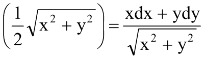# General form of variable separable differential equations

## General form of variable separable differential equations

If we can write the differential equation as f1(f2(x)) d(f2(x)) + f3 (f4(x)) d(f4(x)) + ..... + f2n - 1 (f2n (x)) d (f2n (x)) = 0, where f1, f2,....., f2n are real valued function. Then integrate each term w.r.t f2(x), f4(x), ..... , f2n(x) respectively, we get the solution of the given differential equation for this the following derivatives must be remembered.

(i) d(x + y) = dx + dy (ii) d(xy) = y dx + x dy

(iii) d =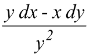(iv) d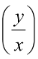=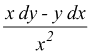(v) d(log xy) =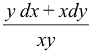(vi) d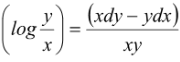(vii) d